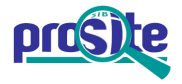Entry: PS00699

 Entry name [info] NITROGENASE_1_1 Accession [info] PS00699 Entry type [info] PATTERN Date [info] 01-DEC-1992 CREATED; 01-NOV-1995 DATA UPDATE; 22-FEB-2023 INFO UPDATE. PROSITE Doc. [info] PDOC00085

Name and characterization of the entry

 Description [info] Nitrogenases component 1 alpha and beta subunits signature 1. Pattern [info] ```[LIVMFYH]-[LIVMFST]-H-[AG]-[AGSP]-[LIVMNQA]-[AG]-C. ```

Numerical results [info]

Numerical results for UniProtKB/Swiss-Prot release 2023_01 which contains 569'213 sequence entries.

 Total number of hits 87 in 87 different sequences Number of true positive hits 79 in 79 different sequences Number of 'unknown' hits 1 Number of false positive hits 7 in 7 different sequences Number of false negative sequences 6 Number of 'partial' sequences 2 Precision (true positives / (true positives + false positives)) 91.86 % Recall (true positives / (true positives + false negatives)) 92.94 %

 UniProtKB/Swiss-ProtTrue positive sequences 79 sequences ```ANFD_AZOVI (P16266 ), ANFD_HELGE (O68955 ), ANFD_RHOCA (Q07933 ), ``` ```ANFD_RUMHU (Q46082 ), ANFK_AZOVI (P16267 ), ANFK_RHOCA (Q07935 ), ANFK_RUMHU (Q46084 ), NIFD_ACIFI (P06662 ), NIFD_ALCFA (Q44045 ), NIFD_AZOBR (P25313 ), NIFD_AZOVI (P07328 ), NIFD_BRADU (P06121 ), NIFD_BRASP (P06120 ), NIFD_CLOPA (P00467 ), NIFD_CROS5 (O07642 ), NIFD_FRAAL (Q02452 ), NIFD_HERSE (P77874 ), NIFD_KLEPN (P00466 ), NIFD_LEPBY (Q00239 ), NIFD_METBA (P55170 ), NIFD_METTL (P20620 ), NIFD_NOSCO (P52337 ), NIFD_NOSS1 (P00464 ), NIFD_RHICP (P06769 ), NIFD_RHILT (P00465 ), NIFD_RHOCA (P08717 ), NIFD_RIPO1 (Q55029 ), NIFD_SINFN (P19066 ), NIFE_AZOVI (P08293 ), NIFE_BRADU (P26506 ), NIFE_CLOPA (P10996 ), NIFE_KLEPN (P08737 ), NIFE_METBA (P51755 ), NIFE_METMI (P0CW54 ), NIFE_METMP (P0CW55 ), NIFE_METTH (O27607 ), NIFE_METTM (P95297 ), NIFE_NOSS1 (Q44144 ), NIFE_RHILO (Q98AP4 ), NIFE_RHIME (Q92ZL2 ), NIFE_RHOCA (P19055 ), NIFE_RIPO1 (O07355 ), NIFE_SINFN (P55673 ), NIFK_ACIFI (P15052 ), NIFK_AZOBR (P25314 ), NIFK_AZOVI (P07329 ), NIFK_BRADU (P20621 ), NIFK_BRASP (P06122 ), NIFK_CLOPA (P11347 ), NIFK_CROS5 (O07643 ), NIFK_FRAAL (Q57118 ), NIFK_HERSE (P77875 ), NIFK_KLEPN (P09772 ), NIFK_METBA (P51754 ), NIFK_METMI (P0CW52 ), NIFK_METMP (P0CW53 ), NIFK_METTH (O27606 ), NIFK_METTM (P95296 ), NIFK_RIPO1 (Q55030 ), NIFK_SINFN (P19067 ), NIFN_AZOVI (P10336 ), NIFN_BRADU (P26507 ), NIFN_HERSE (O87627 ), NIFN_KLEPN (P08738 ), NIFN_NOSS1 (Q44145 ), NIFN_RHILO (Q98AP3 ), NIFN_RHIME (P12781 ), NIFN_RHOCA (P19077 ), NIFN_RIPO1 (O07356 ), NIFN_SINFN (P55674 ), VNFD_AZOCH (P15332 ), VNFD_AZOPA (O68953 ), VNFD_AZOSA (O68954 ), VNFD_AZOVI (P16855 ), VNFK_AZOCH (P15334 ), VNFK_AZOPA (O68949 ), VNFK_AZOSA (O68952 ), VNFK_AZOVI (P16856 ), VNFK_TRIV2 (Q57302 ) ``` » more Retrieve an alignment of UniProtKB/Swiss-Prot true positive hits: [Clustal format, color, condensed view] [Clustal format, color] [Clustal format, plain text] [Fasta format] Retrieve the sequence logo from the alignment UniProtKB/Swiss-ProtFalse negative sequences 6 sequences ```NIFD_METMI (P0CW50 ), NIFD_METMP (P0CW51 ), NIFD_METTH (O27605 ), ``` ```NIFD_METTM (Q50788 ), NIFK_METTL (P20622 ), NIFK_NOSS1 (P00468 ) ``` » more UniProtKB/Swiss-Prot'Partial' sequences 2 sequences ```NIFD_ANASL (P33177 ), NIFD_METIV (P51605 ) ``` UniProtKB/Swiss-Prot'Unknown' sequences 1 sequence ```Y584_MYCTU (O86365 ) ``` UniProtKB/Swiss-ProtFalse positive sequences 7 sequences ```CETP_CHICK (Q3V6R6 ), MDGA1_CHICK (Q0WYX8 ), MDGA1_HUMAN (Q8NFP4 ), ``` ```MDGA1_MOUSE (Q0PMG2 ), MDGA1_RAT (P85171 ), NAGA_VIBFU (P96166 ), Y025_OSHVF (Q6R7J8 ) ``` » more PDB[Detailed view] 62 PDB 1FP4; 1G20; 1G21; 1H1L; 1L5H; 1M1N; 1M1Y; 1M34; 1MIO; 1N2C; 1QGU; 1QH1; » more